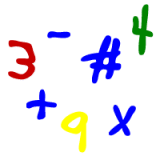Parents and Teachers: Support Ducksters by following us onor.

# Kids Math

## Roman NumeralsSkills needed:
Multiplication
Subtraction

Roman numerals were used by the Ancient Romans as their numbering system. We still use them sometimes today. You see them in the Super Bowl's numbering system, after king's names (King Henry IV), in outlines, and other places. Roman numerals are base 10 or decimal, like the numbers we use today. They are not entirely positional, however, and there is no number zero.

Roman numerals use letters instead of numbers. There are seven letters you need to know:
• I = 1
• V = 5
• X = 10
• L = 50
• C = 100
• D = 500
• M = 1000
You put the letters together to make numbers. Here are a few simple examples:

1) III = 3

three I's together is three 1's and 1 + 1 + 1 equals 3

2) XVI = 16

10 + 5 + 1 = 16

These examples were simple, but there are a few rules and a few tricky things to know when using Roman numerals:
1. The first rule just says that you add letters, or numbers, if they come after a bigger letter or number. We demonstrated this in example 2 above. The V is less than the X, so we added it to the number. The I was less than the V, so we added it to the number. We'll discuss what happens when a letter of greater value comes after a letter of lesser value in rule 3.
2. The second rule is that you can't put more than three letters together in a row. For example, you can put three I's together, III, to make a 3, but you can't put four I's together, IIII, to make a four. How do you make a 4 then? See rule number three.
3. You can subtract a number by putting a letter of lower value before one of higher value.
4. This is how we make the numbers four, nine, and ninety:
• IV = 5 - 1 =4
• IX = 10 - 1 = 9
• XC = 100 - 10 = 90
There are a few restrictions on when you can do this:
• You can only subtract one number. You can't get a 3 by writing IIV.
• You can only do this with I, X, and C. Not with V, L, or D.
• The smaller (subtracted) letter must be either 1/5th or 1/10th the larger one. For example, 99 cannot be written IC because I is 1/100th of C.
5. The last rule is that you can put a bar over a number to multiply it by a thousand and make a really big number.
Examples:

The numbers 1 through 10:

I, II, III, IV, V, VI, VII, VIII, IX, X

The tens (10, 20, 30, 40, 50, 60, 70. 80, 90, 100):

X, XX, XXX, XL, L, LX, LXX, LXXX, XC, C

Take a ten question quiz about Roman numerals.

Advanced Kids Math Subjects

 Multiplication Intro to Multiplication Long Multiplication Multiplication Tips and Tricks Division Intro to Division Long Division Division Tips and Tricks Fractions Intro to Fractions Equivalent Fractions Simplifying and Reducing Fractions Adding and Subtracting Fractions Multiplying and Dividing Fractions Decimals Decimals Place Value Adding and Subtracting Decimals Multiplying and Dividing Decimals Statistics Mean, Median, Mode, and Range Picture Graphs Algebra Order of Operations Exponents Ratios Ratios, Fractions, and Percentages Geometry Polygons Quadrilaterals Triangles Pythagorean Theorem Circle Perimeter Surface Area Misc Basic Laws of Math Prime Numbers Roman Numerals Binary Numbers

Back to Kids Math

Back to Kids StudyFollow us onor